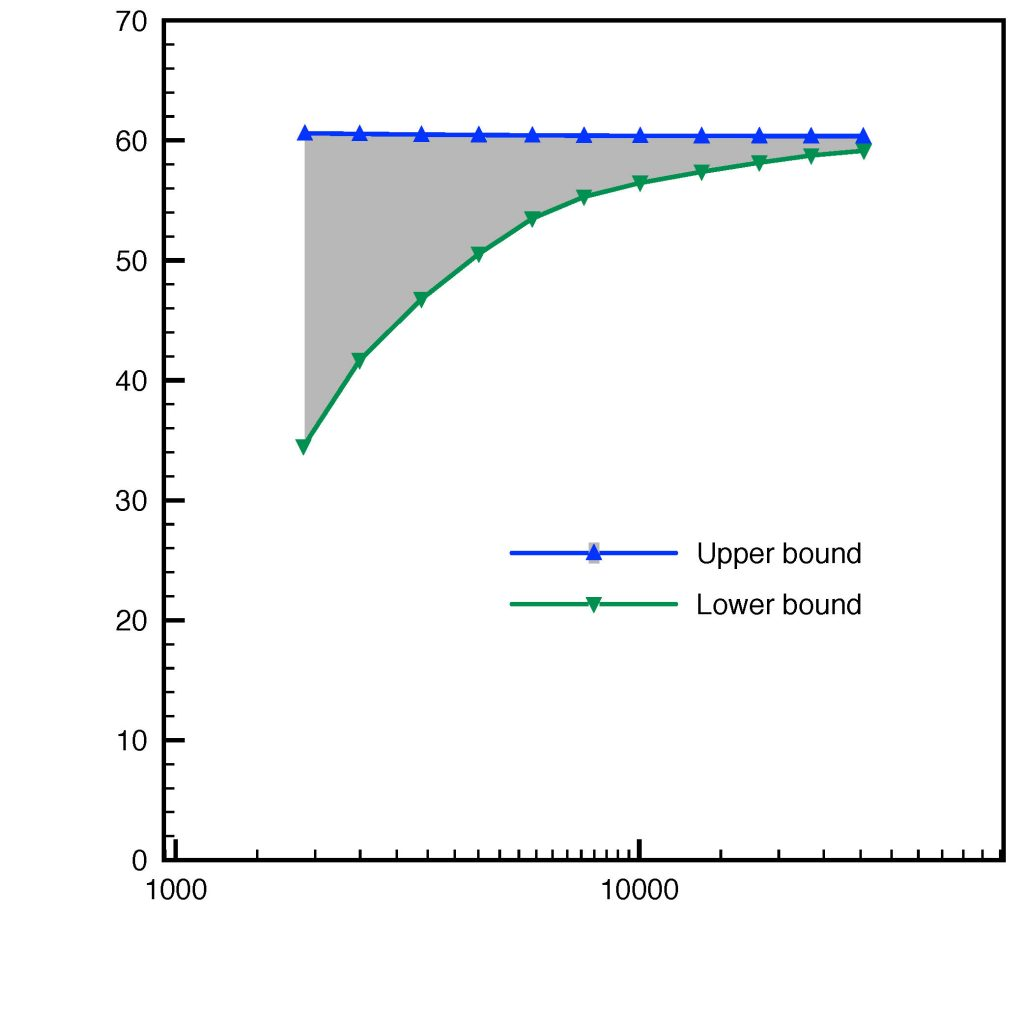# Laplace eigenvalues and eigenvectors boundsFirst eigenvalue and eigenvector bounds on a square (structured mesh)First eigenvalue and eigenvector bounds on a square (unstructured mesh)Inclusion for the first eigenvalue, domain with a hole

Main results:

• guaranteed upper and lower bounds for an arbitrary simple eigenvalue;
• guaranteed energy error bounds on the associated eigenvector;
• efficiency (optimal convergence rate) and polynomial-degree robustness;
• multiplicative constant tending to one under an elliptic regularity assumption on the corresponding
source problem;
• inexact algebraic solvers taken into account;
• unified framework covering basically any numerical method (conforming, nonconforming, discontinuous Galerkin, and mixed finite elements).

Details: conforming setting paper and unified setting paper (see also preprint) with Eric Cancès, Geneviève Dusson, Yvon Maday, and Benjamin Stamm, presentation

Comments are closed.